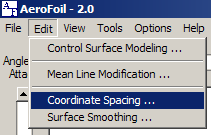## Coordinate SpacingSpacing of the surface coordinates can cause a significant change in the validity of the calculations. Boundary layer development and transition from laminar flow to turbulent flow or separation is highly dependent upon both the fluid velocity and velocity gradient. Sparse coordinate data, especially near the leading edge, will exhibit unrealistic velocity gradients and consequent flow transitions.

In general, additional coordinate data will result in a more realistic velocity gradient; however, location of the coordinates is also important. Best results are obtained by grouping the coordinates more closely at the leading edge. The gradient at the leading edge is highest, especially at higher angle of attack. The calculation of boundary layer thickness and consequent viscous effects are dependent upon the point at which separation occurs. Separation begins at the trailing edge and progresses towards the leading edge as the angle of attack is increased.

Spline calculations are used to find airfoil coordinates in one of two ways. The initial airfoil coordinates are used to generate spline-fit coefficients that are then used to find the new values of the coordinates.

In the "Uniform Spacing" method, coordinates along the leading edge are found at values of x/c given by:
x/c = (1 + Cos θ) / 2
(125 <= θ <= 235 degrees)
which corresponds to the front 20 percent of the airfoil. The rest of the points are evenly spaced as a function of x/c.

The other option is to double the number of points. In this option, the original data points are not altered in any way. Points are added mid-way between each pair.As a rule, even the sparsest set of data may be expanded to the next finer set of values. This routine does not include any smoothing algorithm. Sparse coordinate data that is expanded too far can produce a less than perfect result.